# NCERT Solutions for Class 7 Maths Chapter 9 – Rational Numbers

Class 7 Maths NCERT Solutions Chapter 9 – Rational Numbers comprises of the 2 Exercises

### NCERT Solutions for Class 7 Maths Chapter 9 Exercise 9.1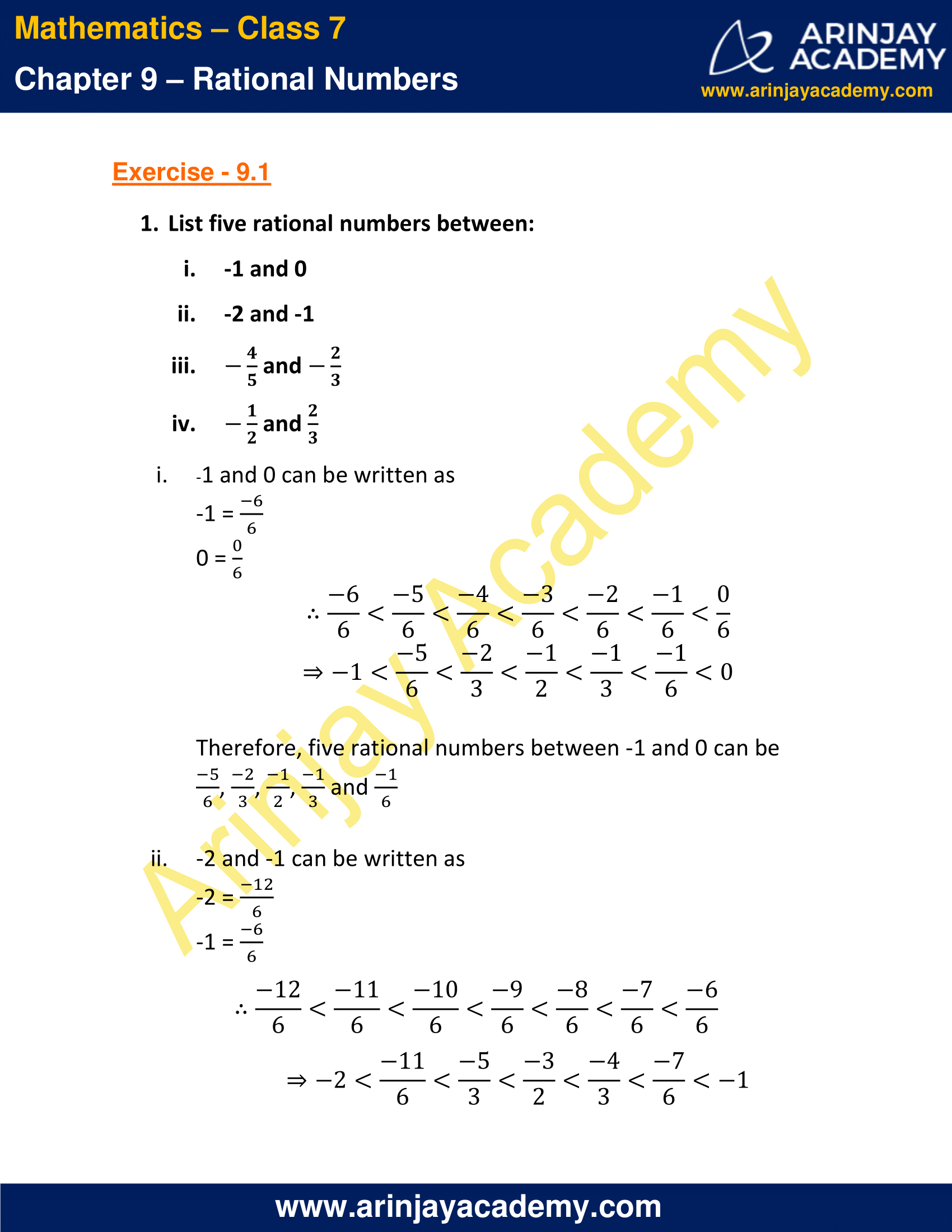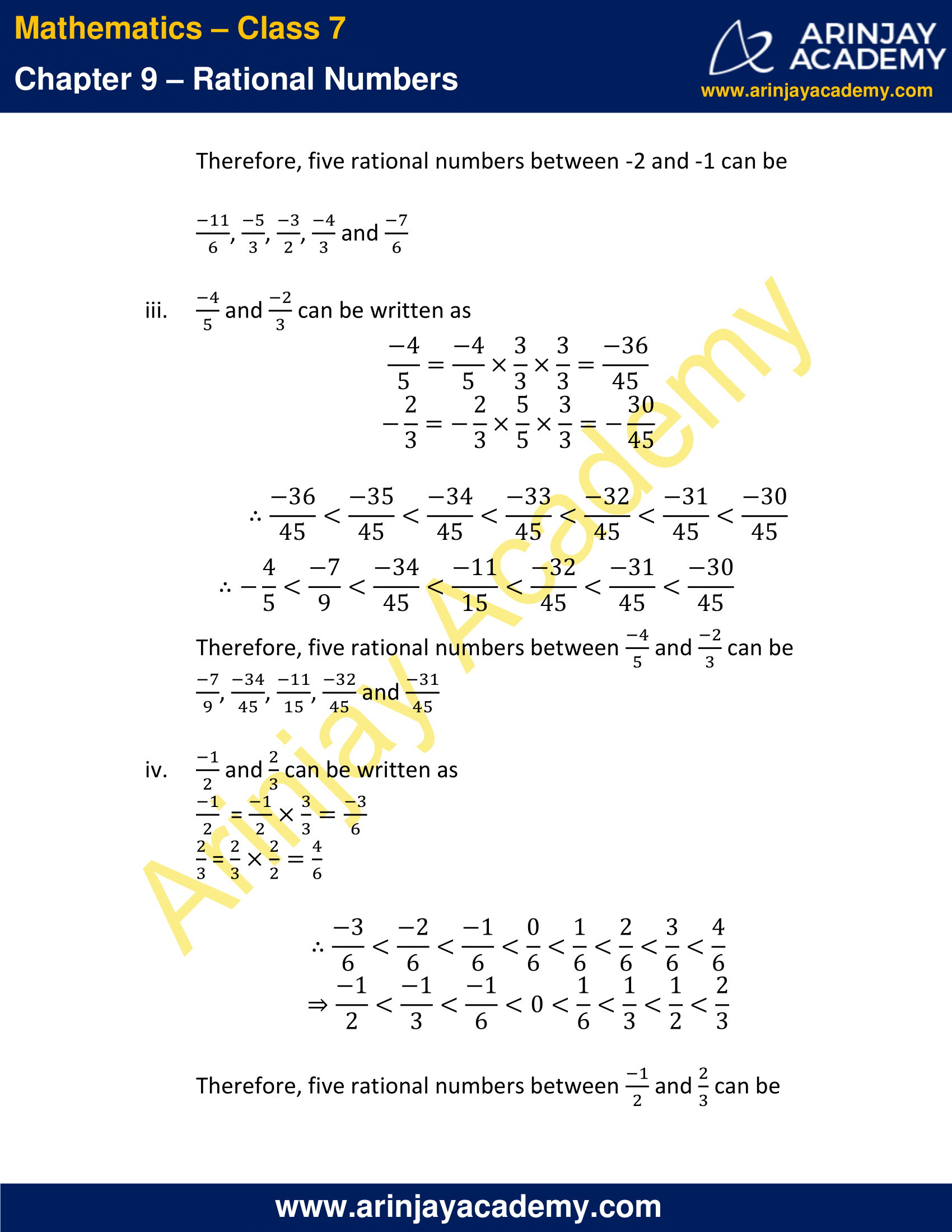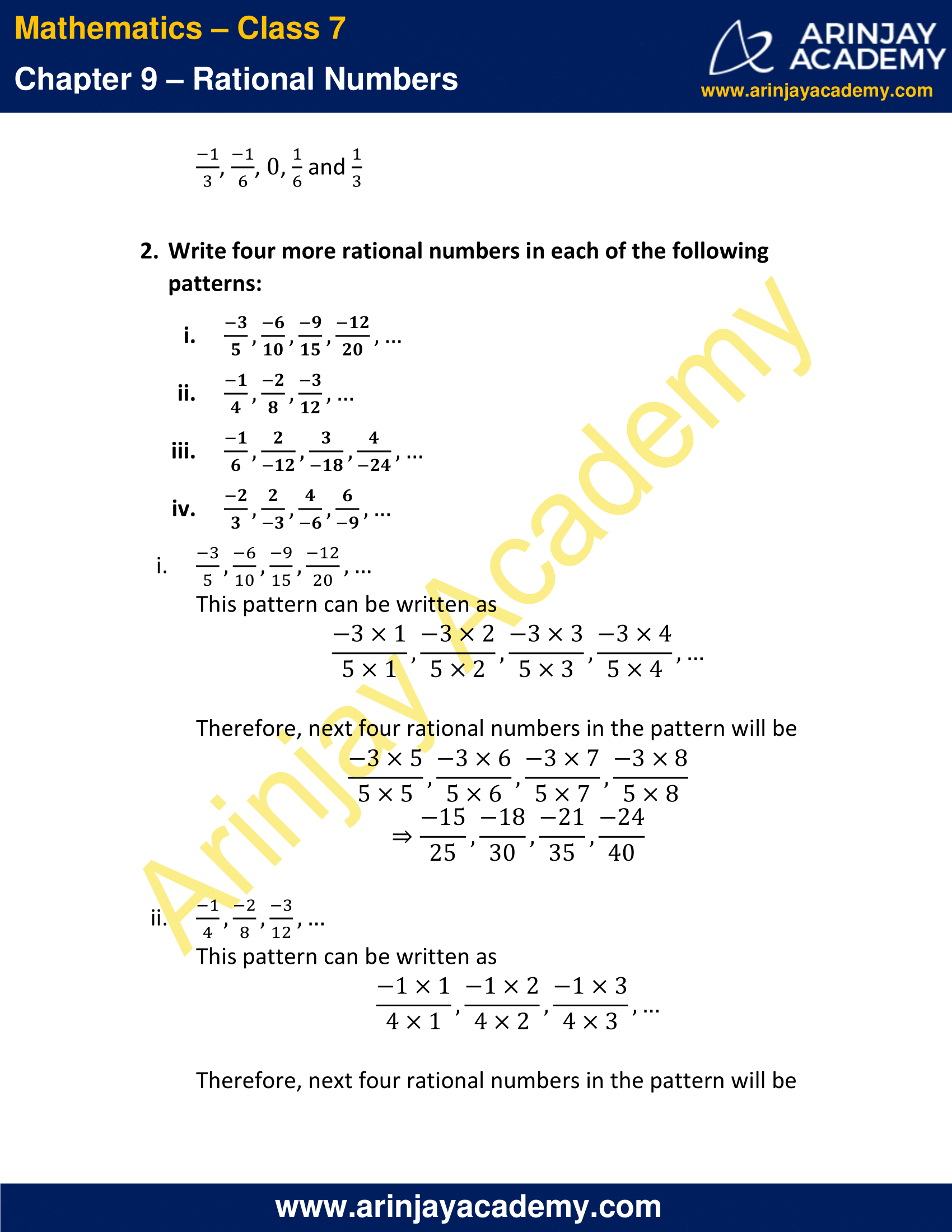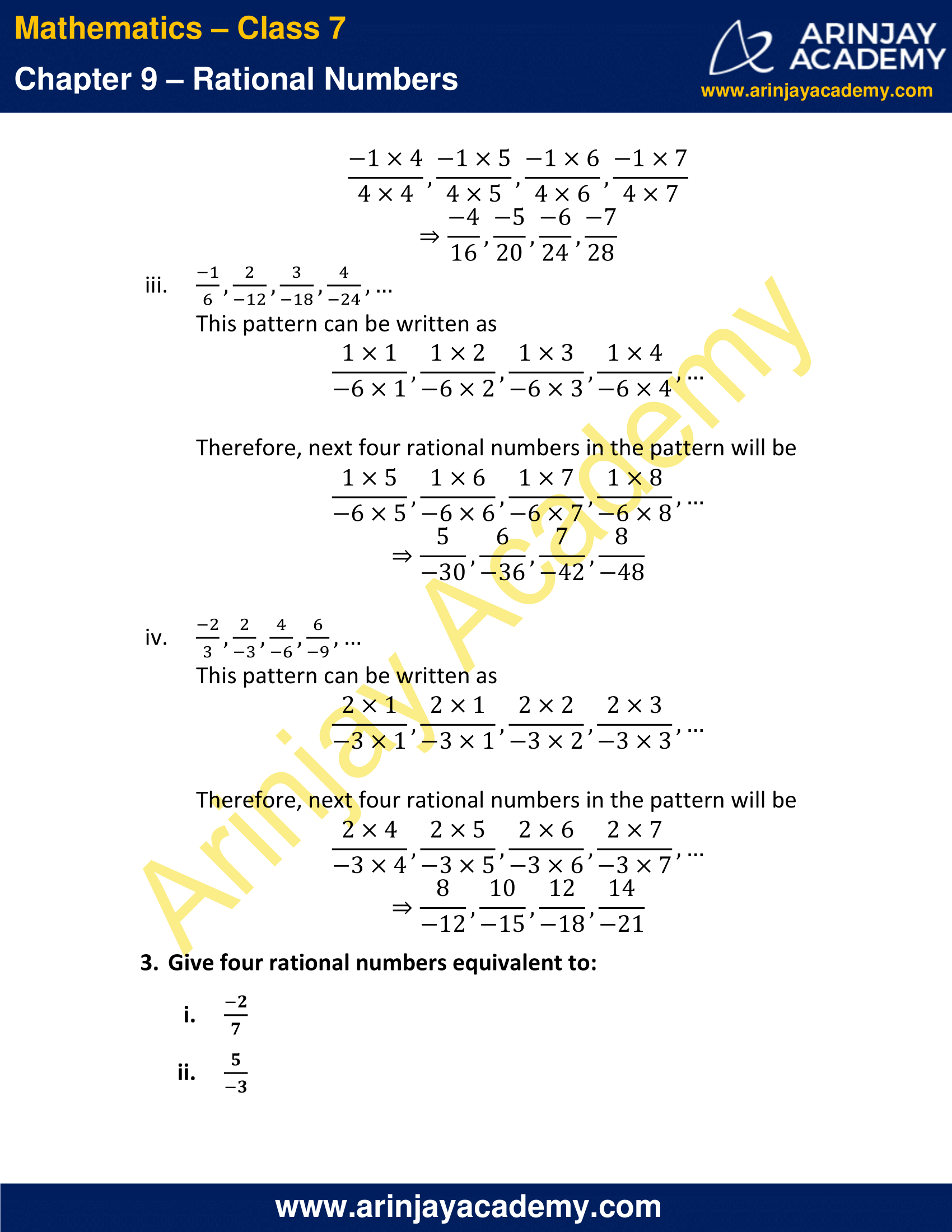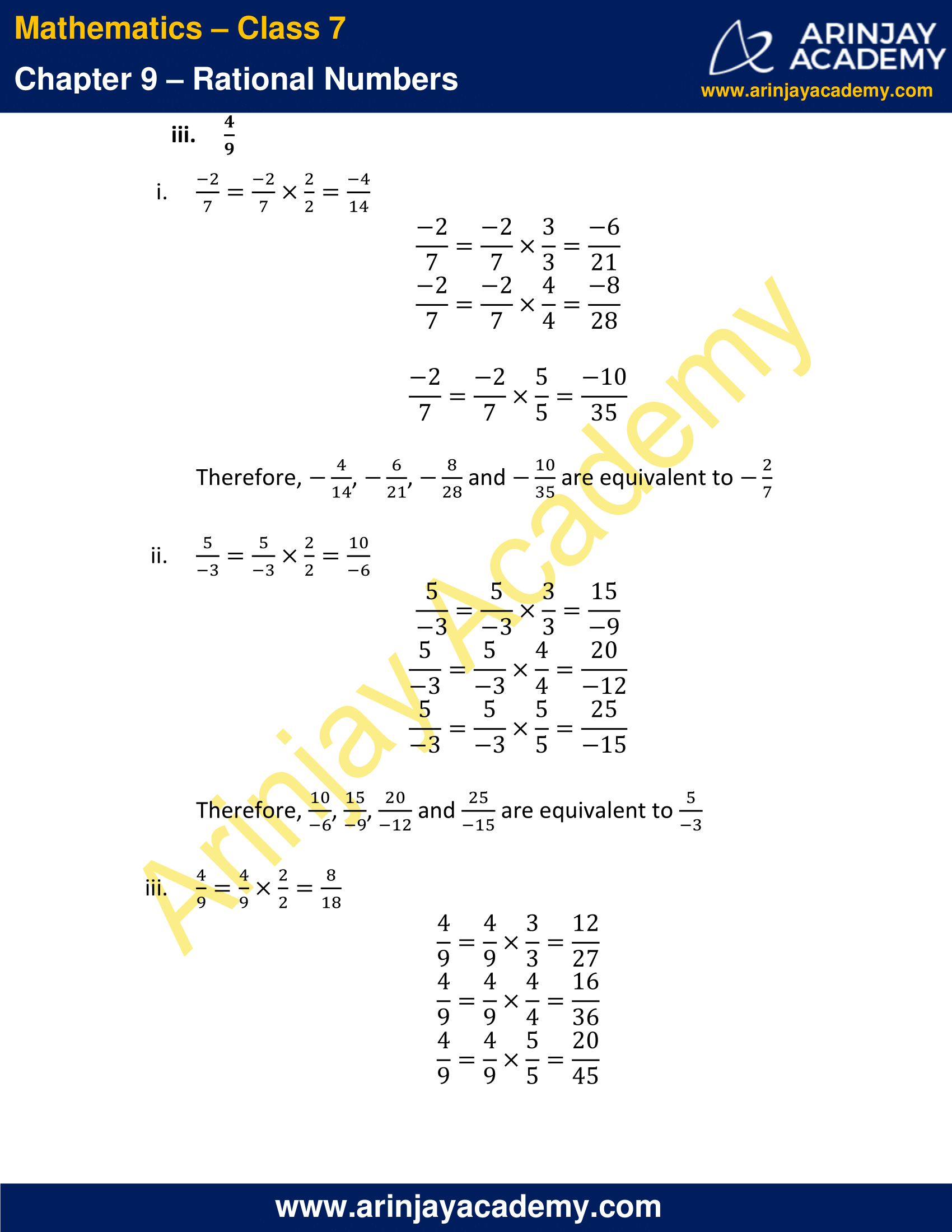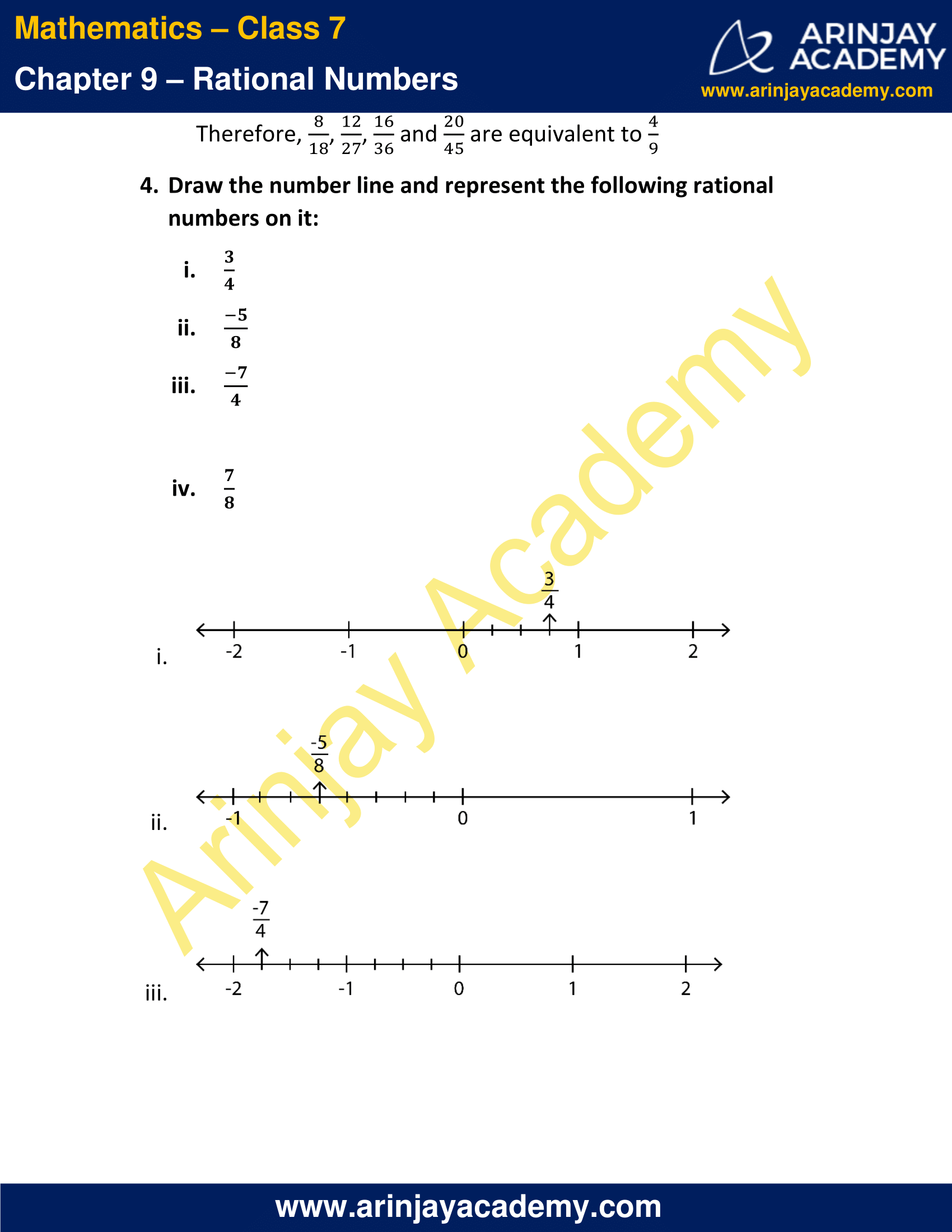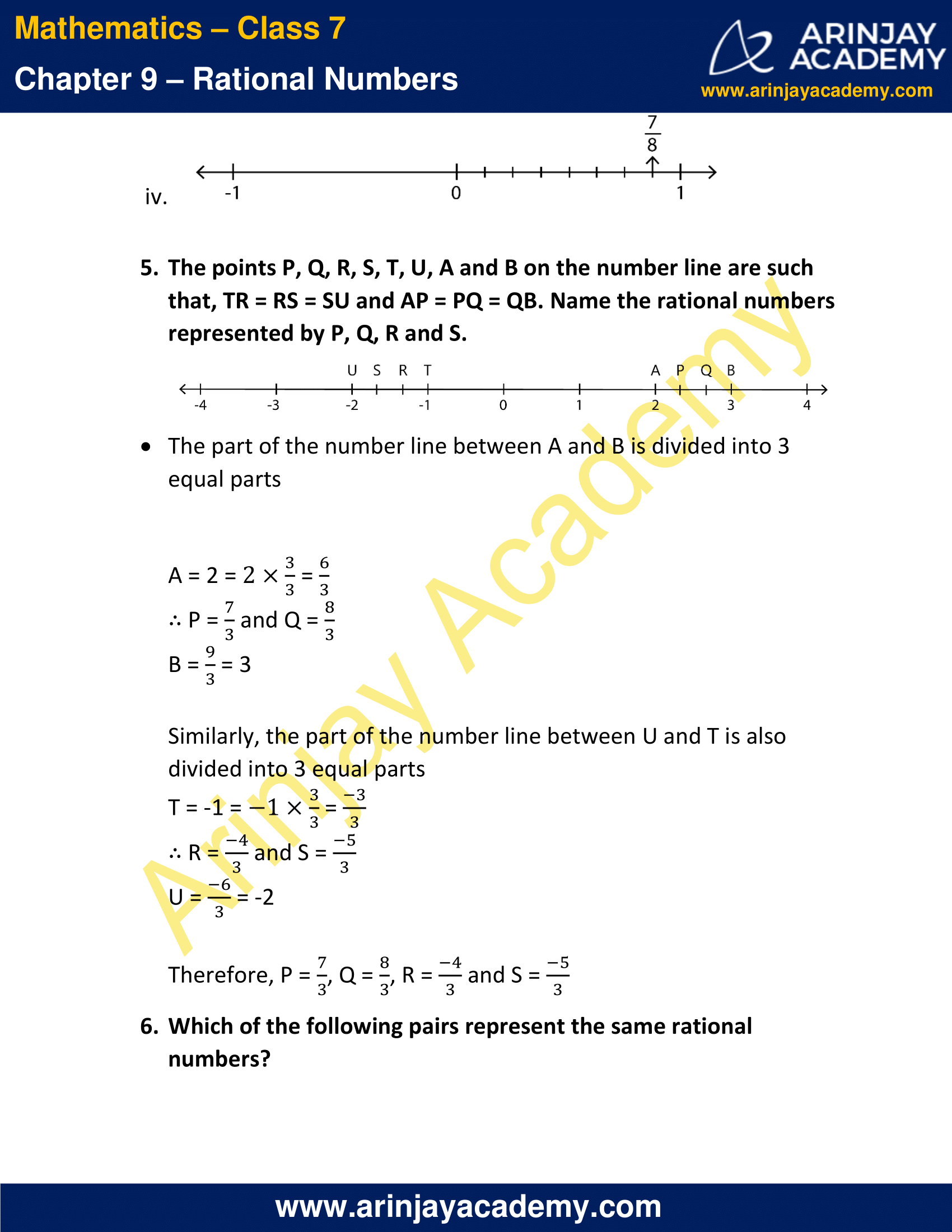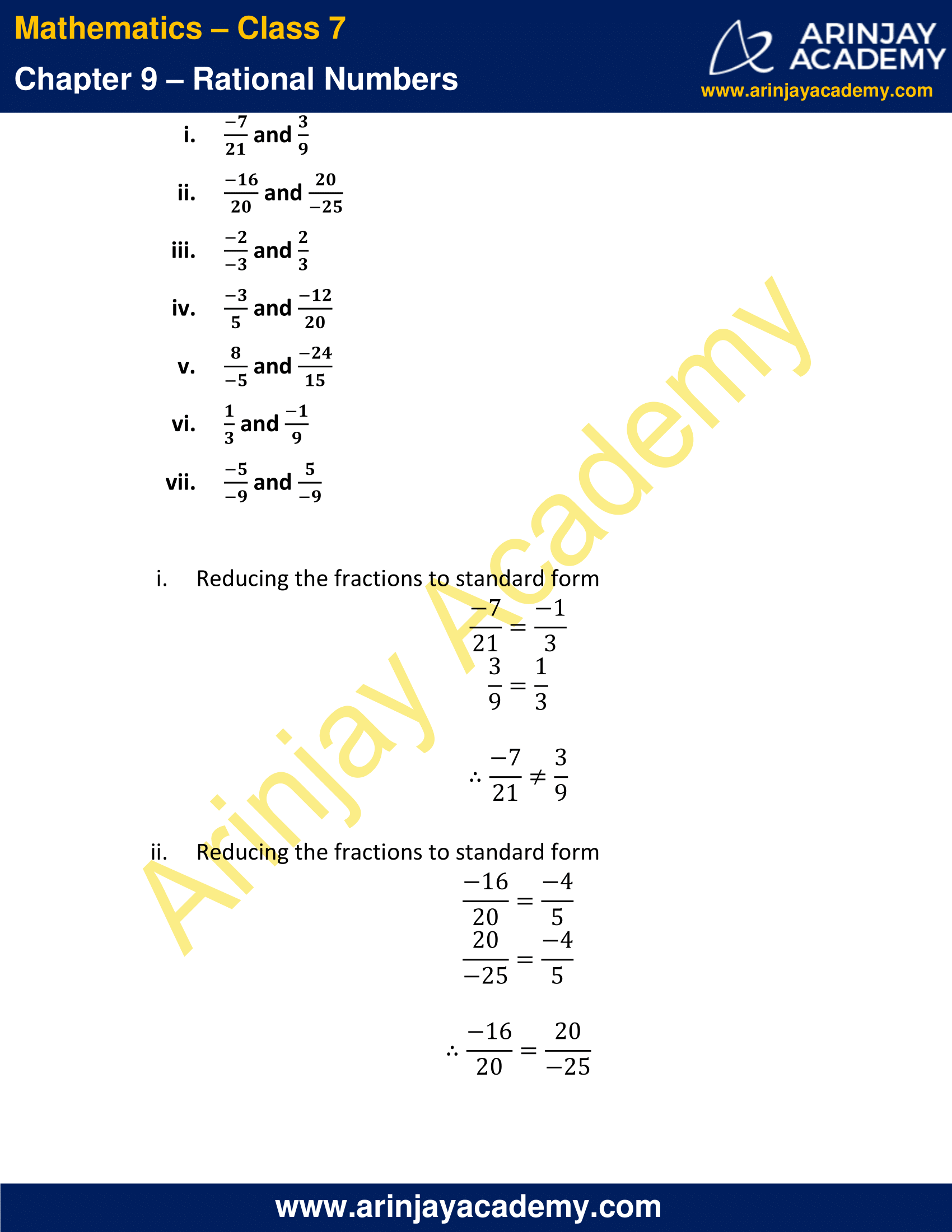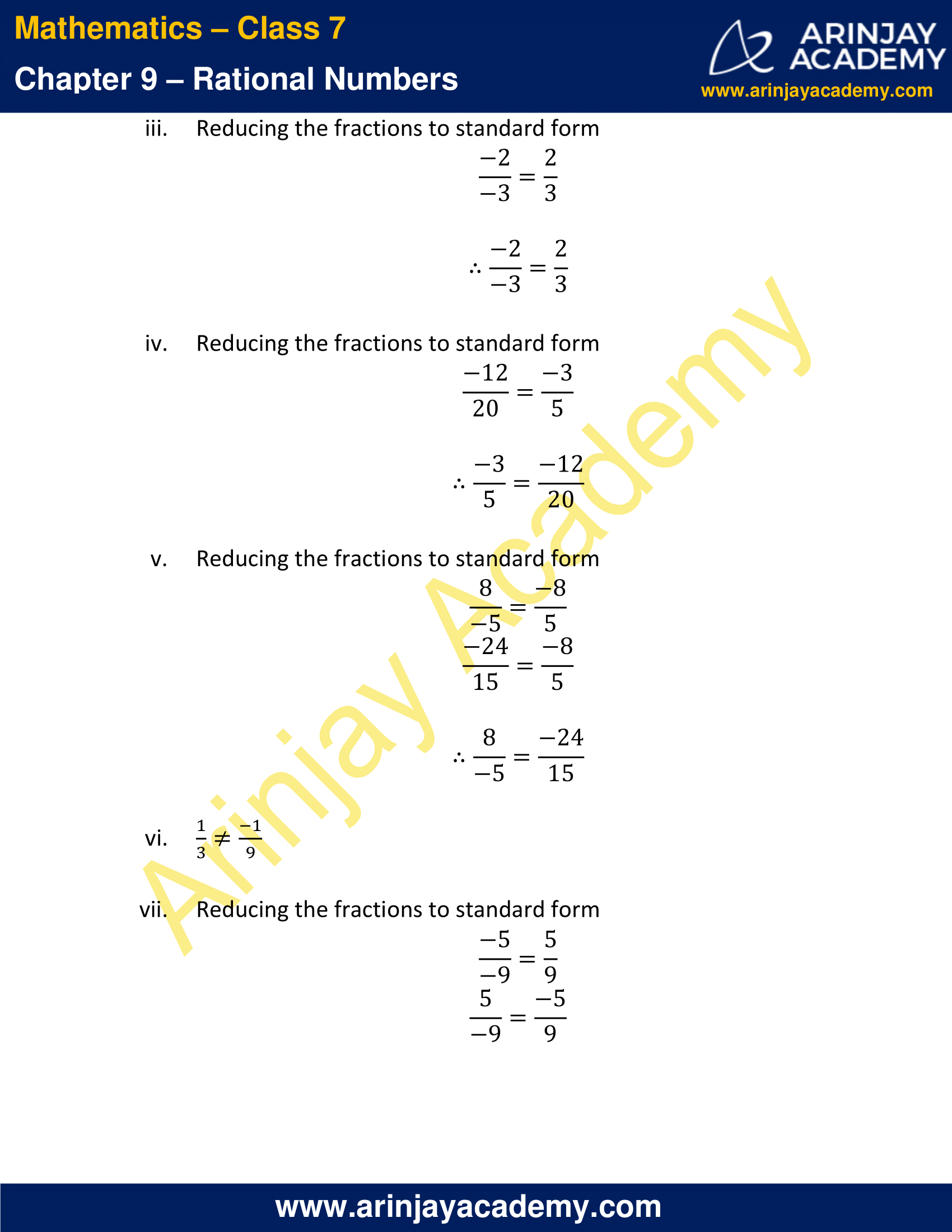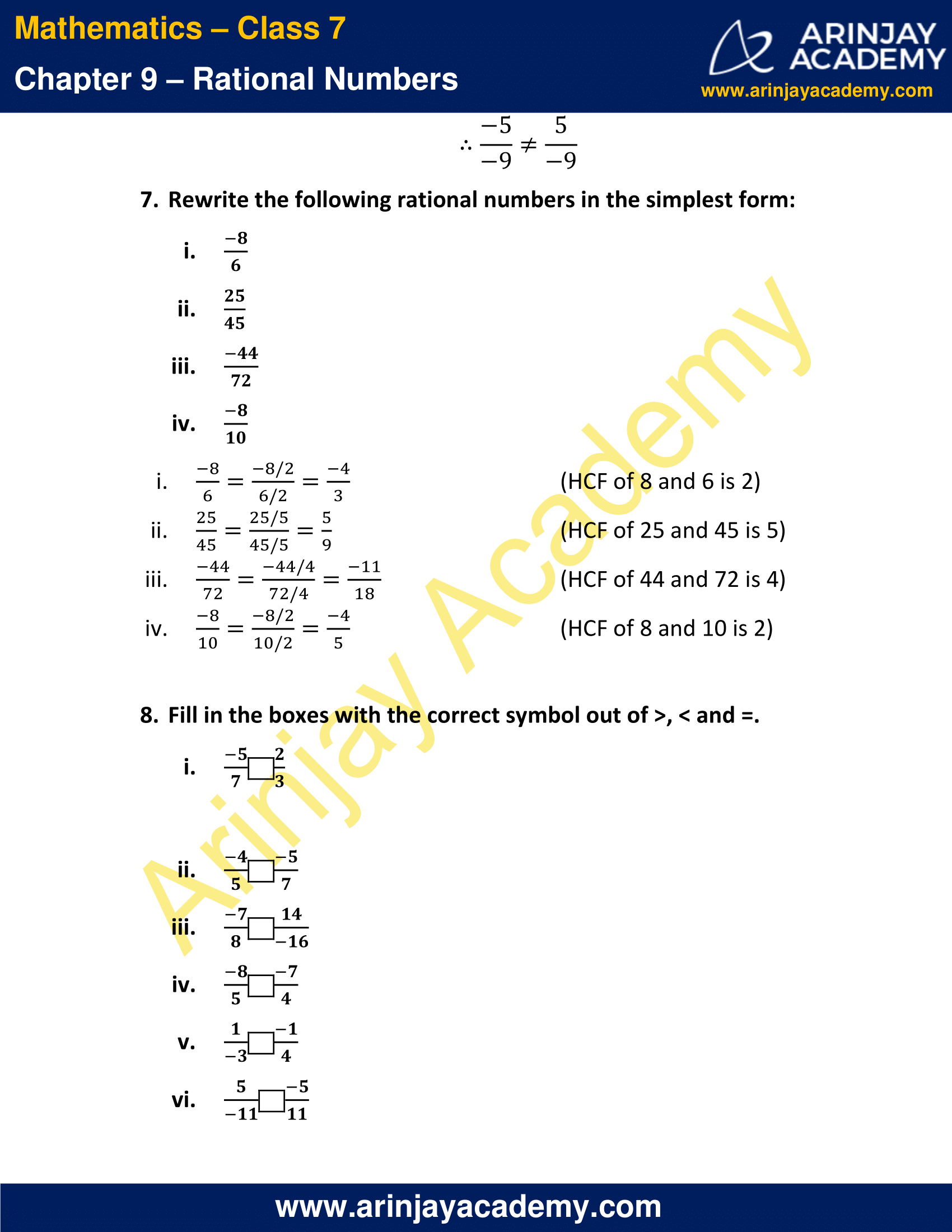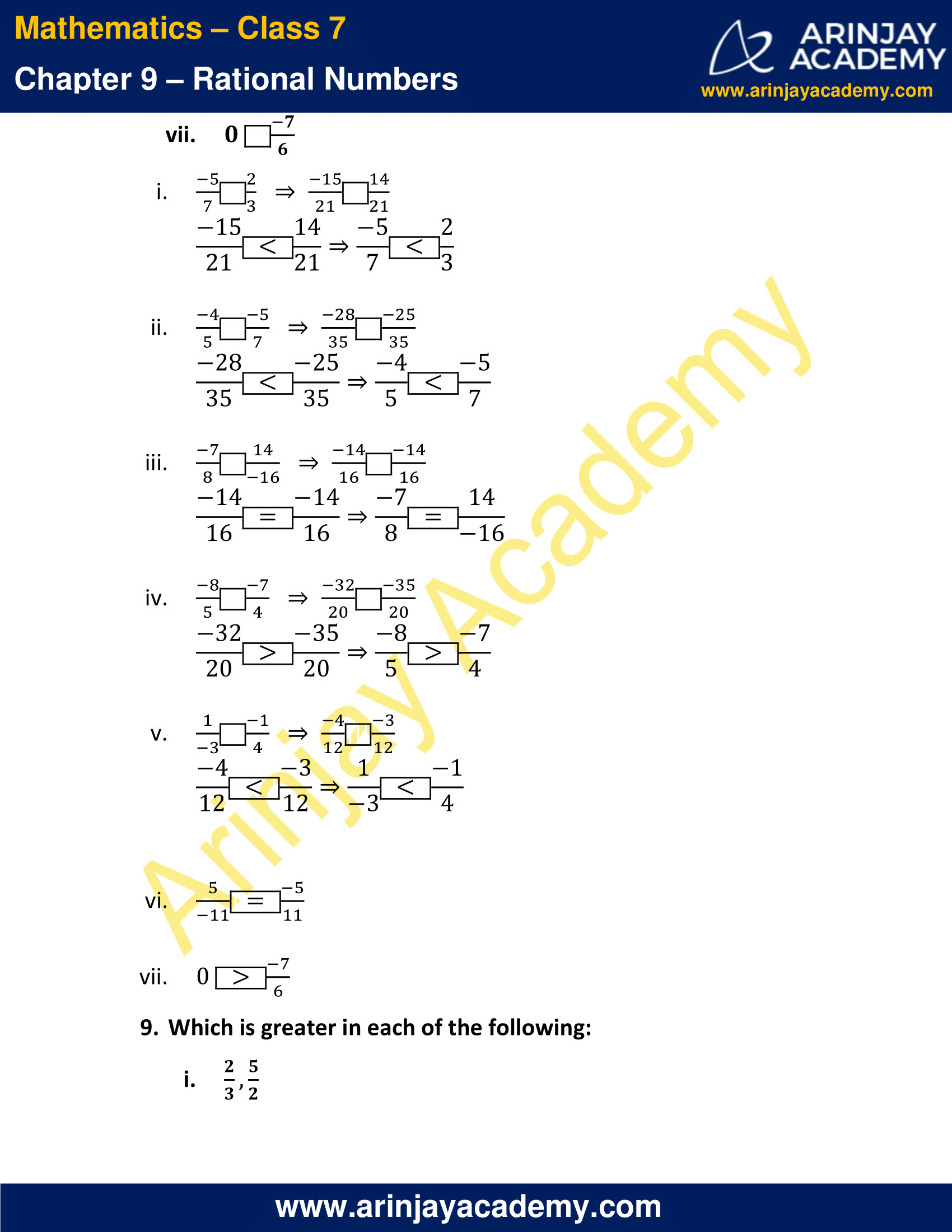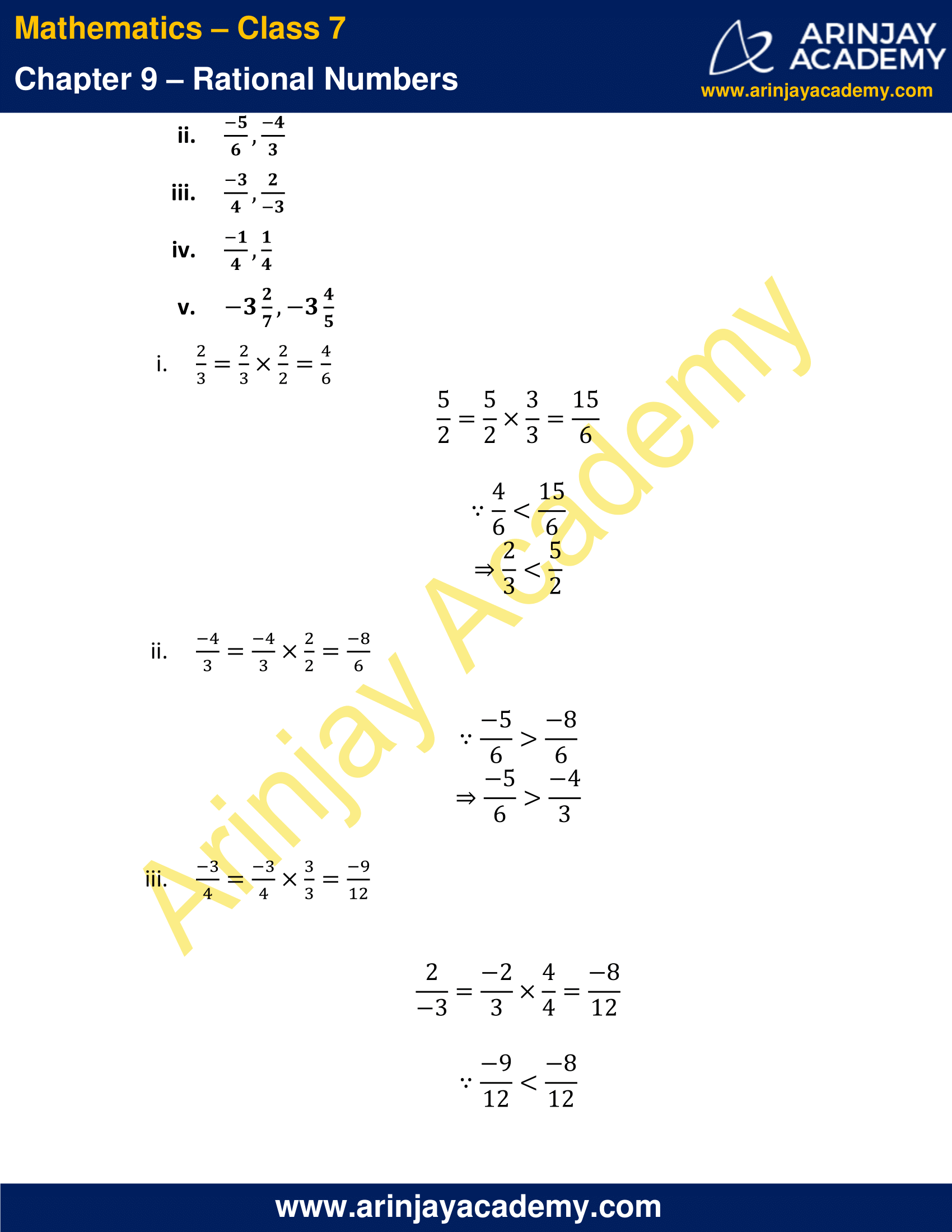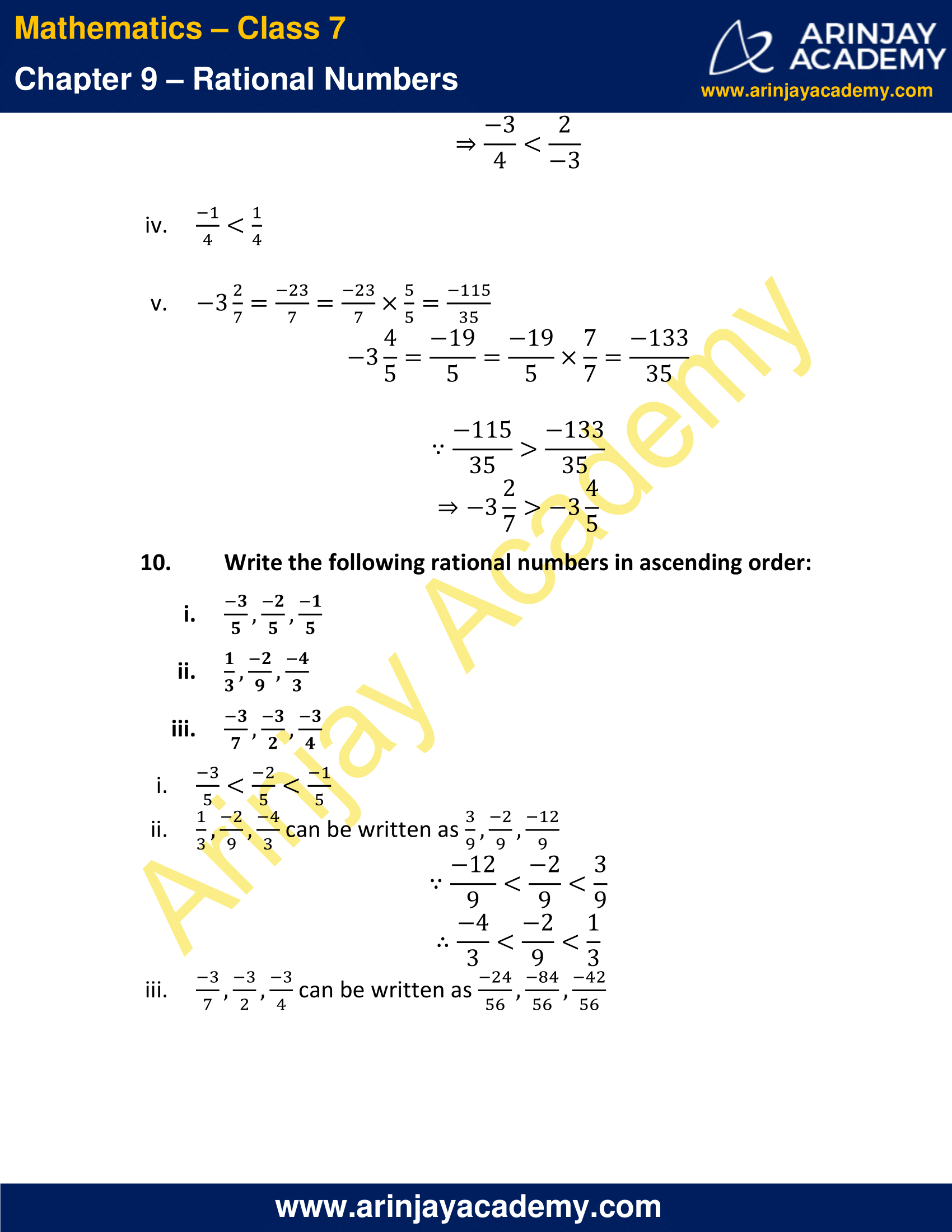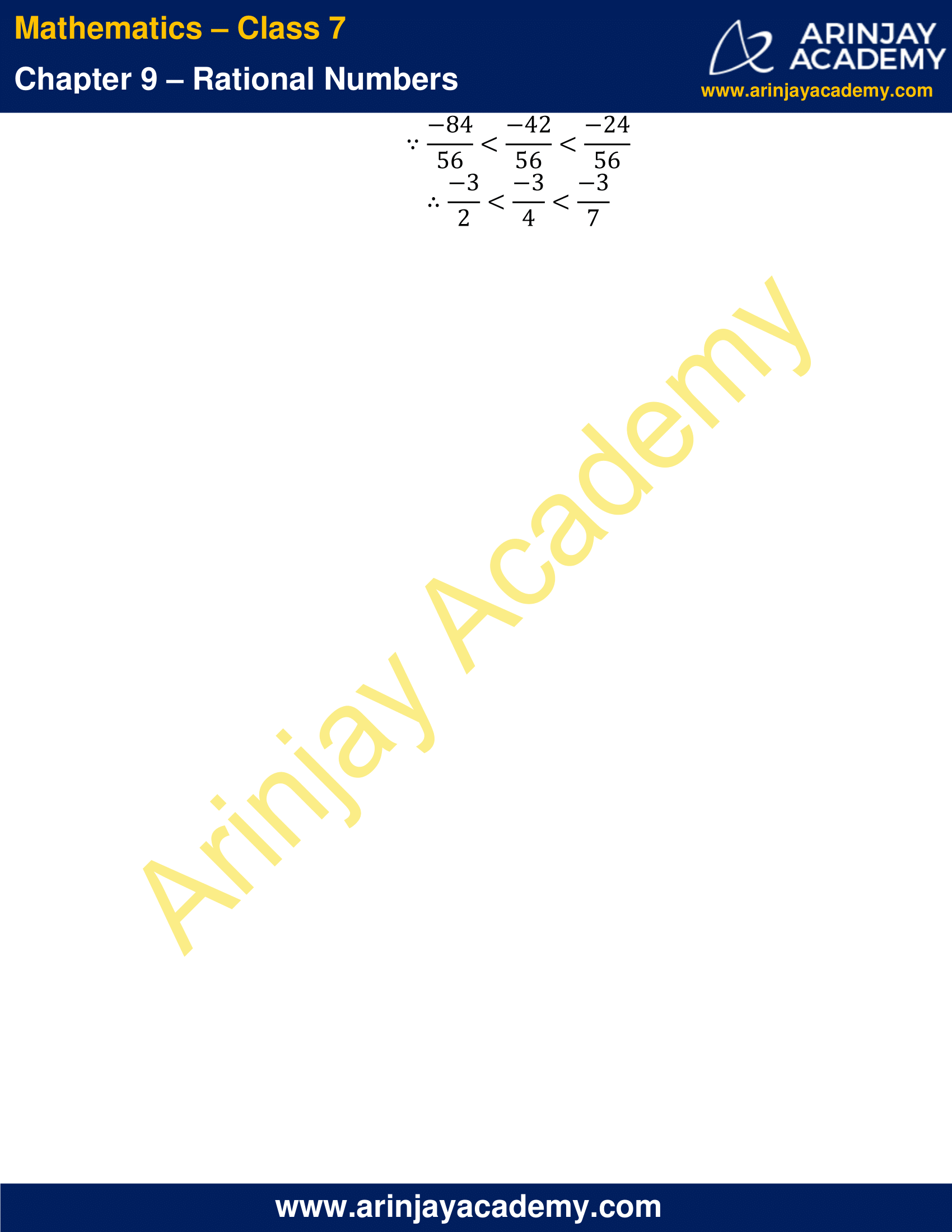### NCERT Solutions for Class 7 Maths Chapter 9 Exercise 9.2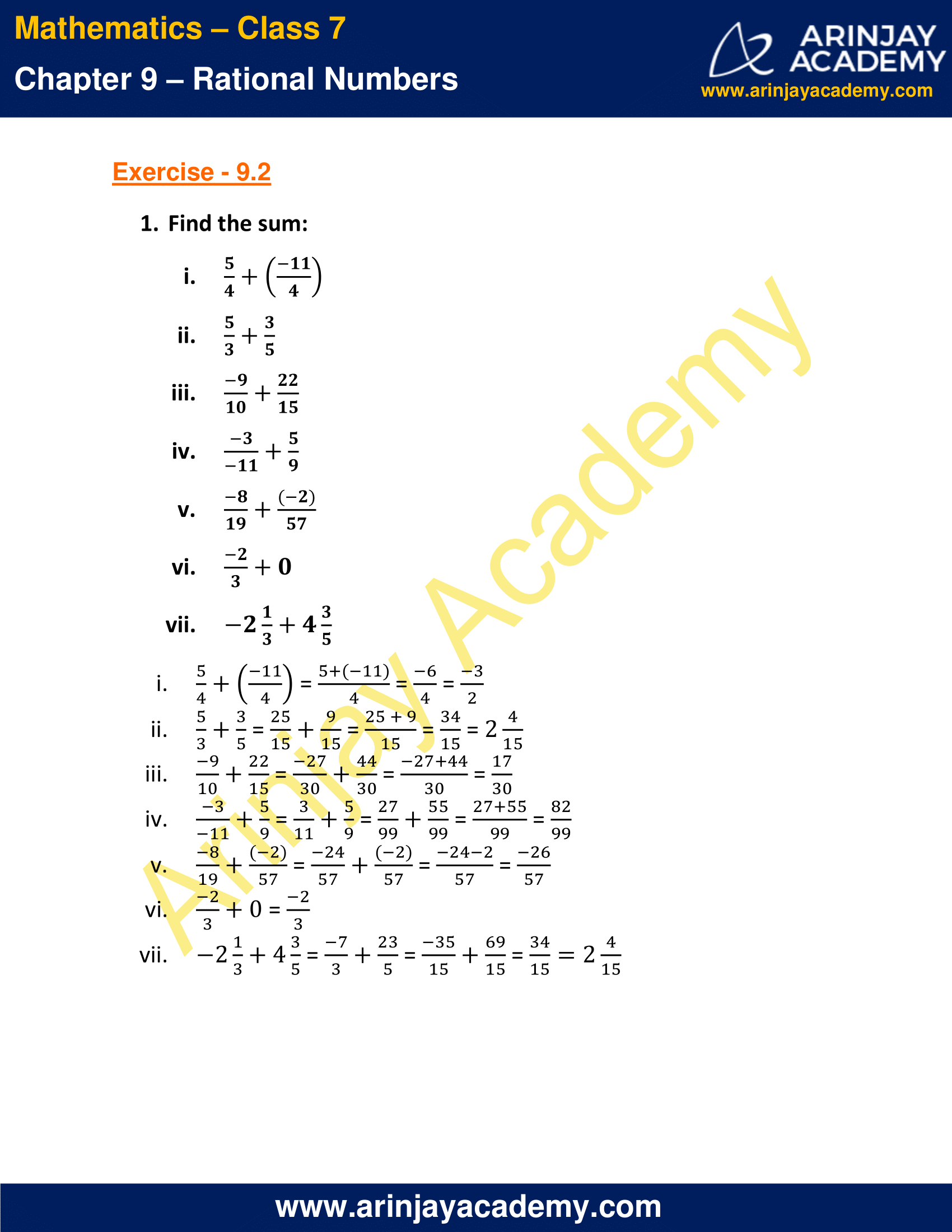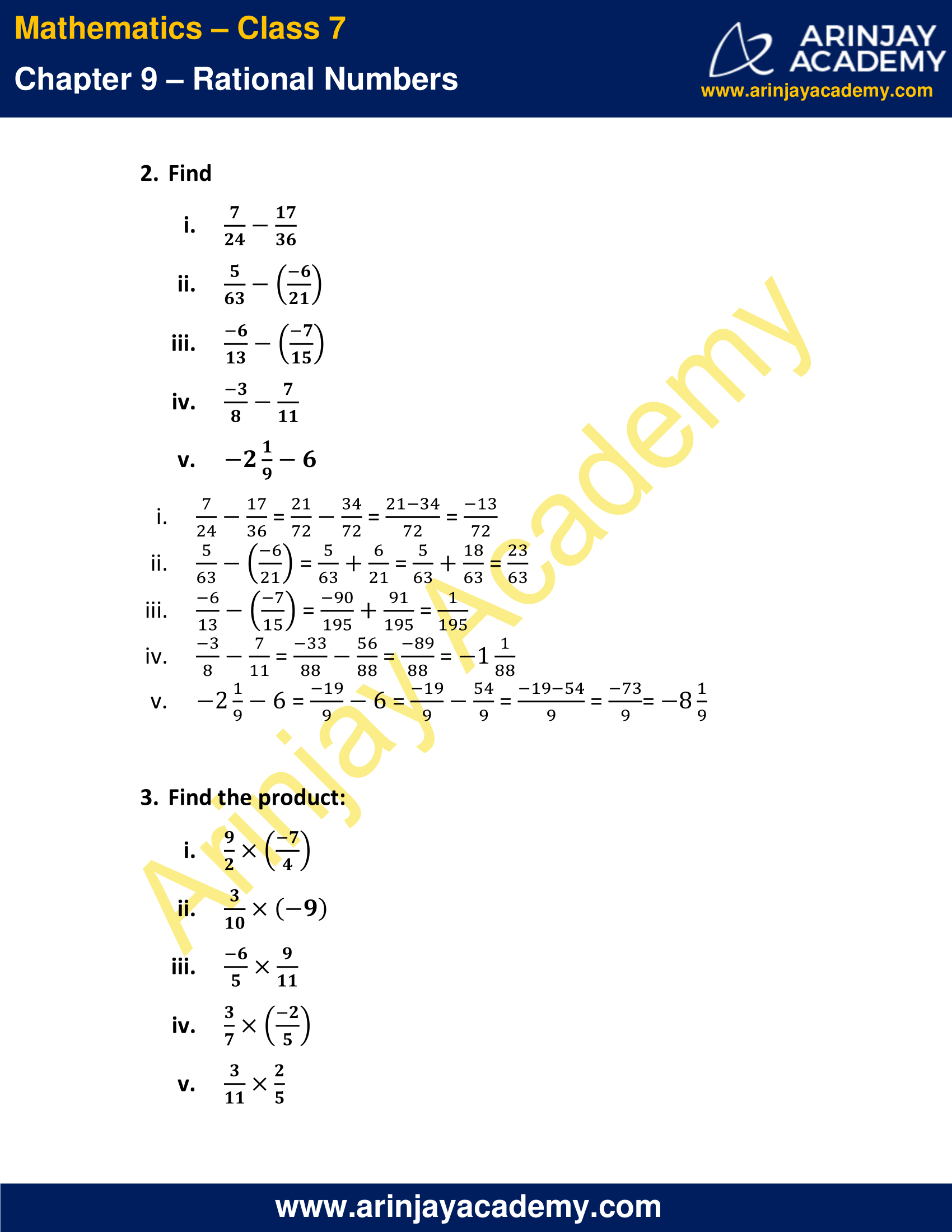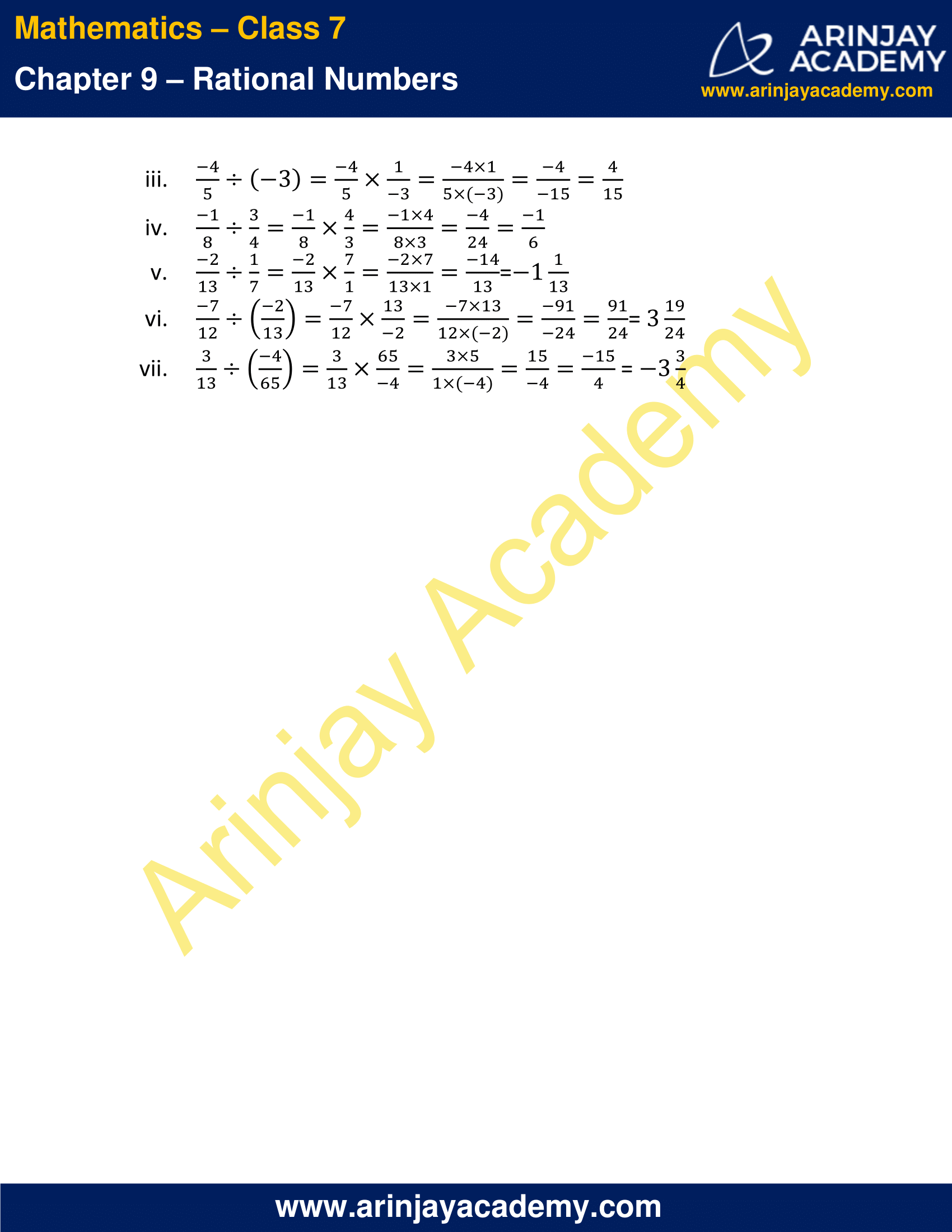This Chapter contains the Exercises relating to the following topics , which are discussed in Chapter 9 – Rational Numbers Class 7 NCERT book  : –

• 9.1 INTRODUCTION
• 9.2 NEED FOR RATIONAL NUMBERS
• 9.3 WHAT ARE RATIONAL NUMBERS?
• 9.4 POSITIVE AND NEGATIVE RATIONAL NUMBERS
• 9.5 RATIONAL NUMBERS ON A NUMBER LINE
• 9.6 RATIONAL NUMBERS IN STANDARD FORM
• 9.7 COMPARISON OF RATIONAL NUMBERS
• 9.8 RATIONAL NUMBERS BETWEEN TWO RATIONAL NUMBERS
• 9.9 OPERATIONS ON RATIONAL NUMBERS